# Orbital Period Calculations

*This post re-published & paraphrased from cache – September 30th 2009*

Since this is something that I recently had to do for a game that I’m a developer on, I thought it would be prudent to put together a write-up on the process of determining orbital period of a body around a central mass; specifically sidereal period.  Overall, this is a fairly simple and straight forward problem, but, even so, it is certainly one that every Physics and Astronomy major and enthusiast should be familiar with.

Now, it should be noted that if an object of significant mass is orbiting a central mass, it is probably a good idea to include both masses in the calculation since it could change the result somewhat significantly.  In order to perform this calculation we need to know the following:

•, the gravitational constant
•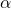, semi-major axis (orbital radius or distance from object 1’s center to object 2’s center)
•, the mass of the central object
•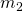, the mass of the orbiting object

So, with this information we can derive a formula from Kepler’s Third Law of motion; the square of the orbital period of a planet is directly proportional to the cube of the semi-major axis of it’s orbit.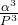and Newton’s Law of Universal Gravitation; attractions are inversely proportional to the squares of the distances between massive bodies.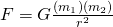Now, the formulas need to be combined to achieve the following relationship: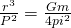The resultant formula can now be manipulated into solving for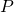or the period of the orbit as follows:Important Note: Since we are using the total mass of the combined bodies we do not multiply the masses for this equation, if calculating the period for 2 large bodies the masses should be added together.

Now that we have our formula, it’s time to work through our information.  The central star is a Type A IV star at ~10,000K with a mass of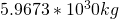.  The planet has a mass of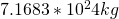and an orbital radius of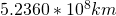.  Next we take this information, along with the Gravitational Constant (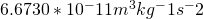and work through our formula:Now combine values and convert the orbital radius into meters so our units match: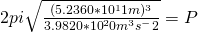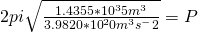Now solve the rest: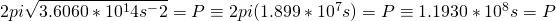Great! Now we have our answer in seconds which means we can convert this number into years to obtain the yearly orbital comparison (the typical way to express orbital periods when it comes to astronomy).  To do this we take our value, divide by 60 for the 60 seconds in a minute, by 60 again for minutes in an hour, by 24 for the hours in a day and then 365 for the days in a year.

[/math]1.1930*10^8s/60s/60m/24h/365d = 3.7830 years[/math]

And there you have it, our answer for the orbital period of the planet is 3.7830 times longer than that of Earths, or 3.7830 years.

In working through this I think there are a few common problems that can happen, so if you attempt this calculation and do not arrive at an answer you would expect, make sure to check the following things:

• Make sure orbital period gets converted into meters at some point.  The Gravitational Constant is calculated in meters which means your orbital radius value should be as well.
• Make sure to add the masses of the bodies.  This is important to note because other similar formulas (such as in calculating weight on another planet) masses are multiplied.  This calculation uses total combined mass.
• Check your units.  If your calculations are complete but you did not net up with seconds for a unit your calculations could be flawed.

In closing, please let me know if you find any flaws with this write-up.  I have put it together carefully, however, I am not immune to mistakes and have corrected several so far.